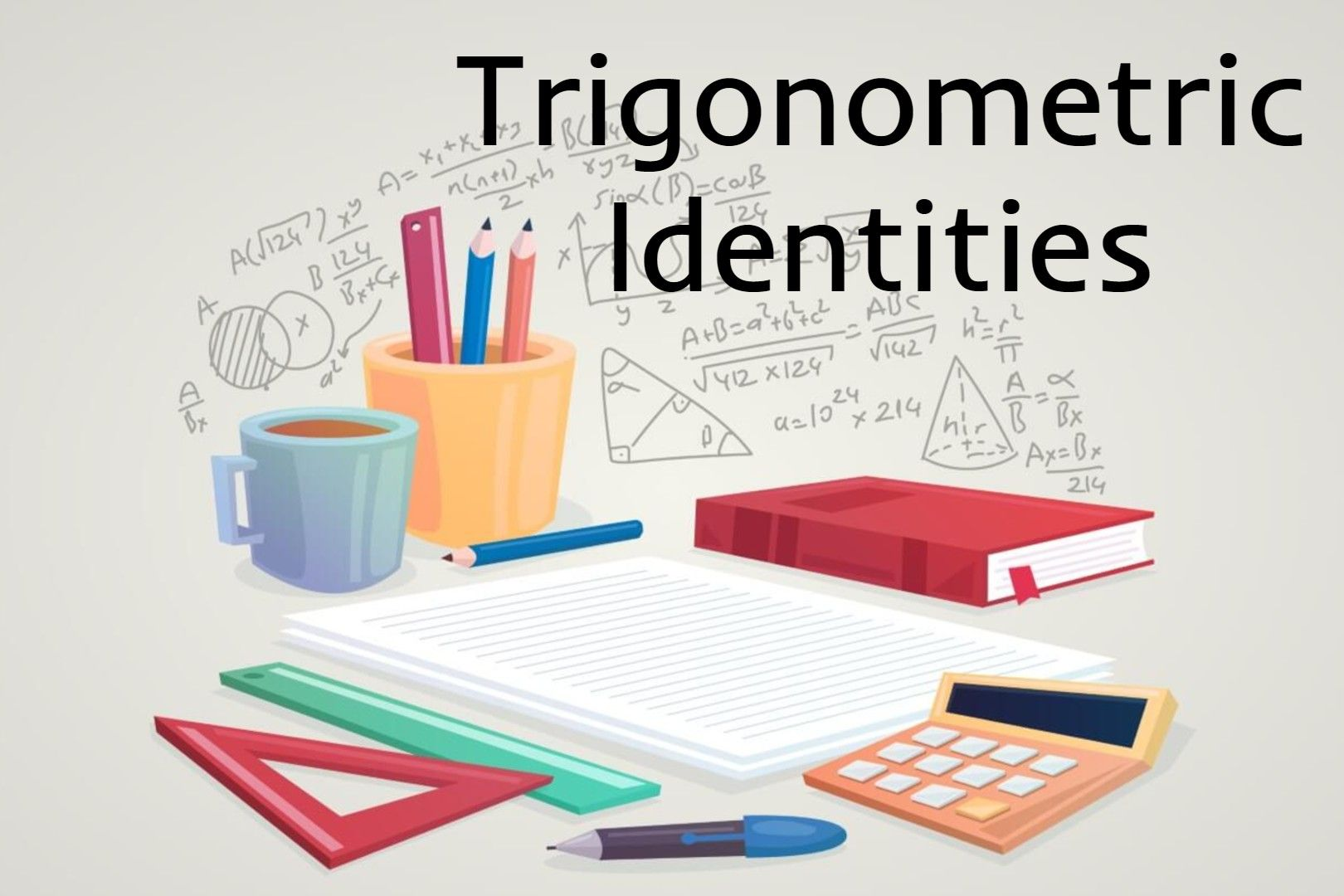## SETS & PROBABILITY## Trigonometric Identities

A Trigonometric identity or trig identity is an identity that contains the trigonometric functions sine(sin), cosine(cos), tangent(tan), cotangent(cot), secant(sec), or cosecant(csc). Trigonometric identities can use to: Simplify trigonometric expressions. Solve…## Role of Euclidean Geometry in High-School Mathematics

If only because in one’s “further” studies of mathematics, the results (i.e., theorems) of euclidean geometry appear only infrequently, this subject has come under frequent scrutiny, especially over the past…